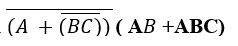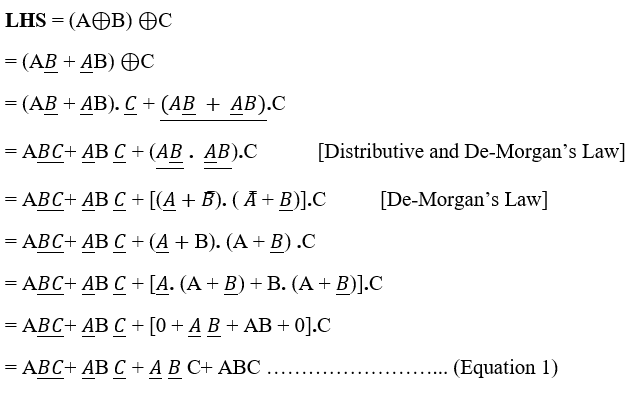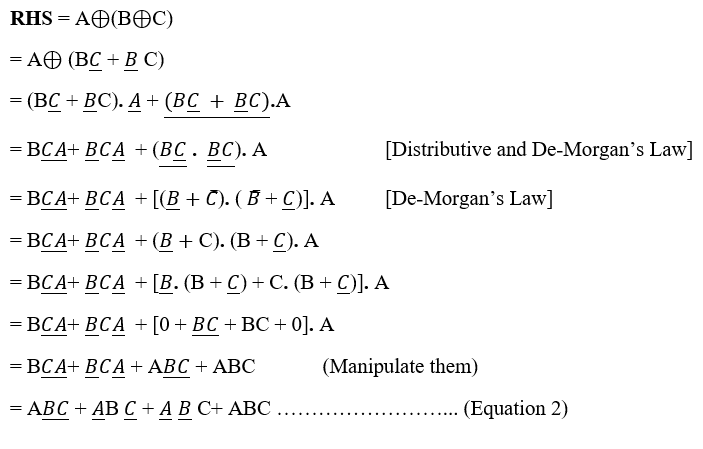# Solved Examples on Reduction of Boolean Expression

Examples on Reduction of Boolean Expression: Here, we have set of some of the Solved Examples on Reduction of Boolean Expression.
Submitted by Saurabh Gupta, on November 18, 2019

Example 1: Simplify the given Boolean Expression to minimum no. of variables or literals.

1. (A+B). (A+B)
2. ABC + AB + ABC

1) (A+B). (A+B)

```    = A.A + A. B + B.A + B.B
= A + A. B + B.A + 0
= A (1 + B + B) = A
```

2) ABC + AB + ABC

```    = ABC + ABC + AB
= AB (C + C) +  AB
= AB +  AB
= B (A + A) = B. 1 = B
```

Example 2: Prove that: (A + C) (A.B +C) = 0

Solution:

```    LHS     = A.(A + C) (A.B + C)
= (A. A + AC) (A.B + C)
= AC (A.B + C)		[Since, A.A = 0]
= AC. A.B + AC. C
= 0 = RHS			[Since, A. A = 0 and C. C = 0]
Hence, our result is proved.
```

Example 3: Reduce the expression (B +BC) (B + BC) (B + D)

Solution:

```    = (B +BC) (B + BC) (B + D)
= (BB +BBC) (B + D)
= (B +0) (B +D)		[Since, B.B =0]
= BB +BD			[Since, B.B =1]
= B + BD
= B (1+D) =B 		[Since, 1+D =1].
```

Example 4: Reduce the expressionSolution:```    = A.BC. (AB +ABC)		        [De-Morgan's Law]
= A.BC (AB+ABC)
= A.BC. AB + A.BC. ABC
= A.A.B.B.C + A.A.B.B.C.C		[Manipulation]
= 0 + 0 = 0
```

Example 4: Prove the Associative Law for XOR operation.

Solution:

We know that XOR operation is given as: AB = AB + AB

To prove associativity for XOR operation we are required to prove:

(AB) C =A(BC). Therefore,From equation 1 and 2 we can see LHS = RHS, thus associative law holds true for XOR operation.